﻿ 纵向数据与生存数据的联合模型—基于机器学习方法 The Joint Model of Longitudinal and Survival Data—Based on Machine Learning Methods

Statistical and Application
Vol.04 No.04(2015), Article ID:16580,10 pages
10.12677/SA.2015.44028

The Joint Model of Longitudinal and Survival Data

—Based on Machine Learning Methods

Zheng Wen

School of Mathematics, Yunnan Normal University, Kunming YunnanReceived: Dec. 2nd, 2015; accepted: Dec. 20th, 2015; published: Dec. 23rd, 2015ABSTRACT

In this paper, machine learning methods for longitudinal data and survival data modeling, replace the longitudinal sub-model linear random effects model; survival sub-model still uses Cox proportional hazards model. Compared with the traditional method, the residuals plots of survival sub- model diagnose modeling methods in line with theoretical results and the residuals of the longitudinal sub models are more dispersed than the linear mixed model.

Keywords:Joint Model, Machine Learning, Martingale Residuals, Cox-Snell Residuals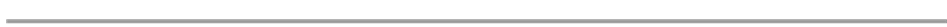—基于机器学习方法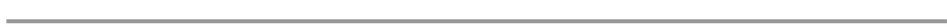1. 引言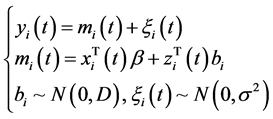(1)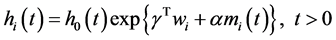(2)

2. 建立模型

2.1. 数据来源及R软件介绍

2.2. 建立模型

1) 传统方法

2) 机器学习方法Table 3. The results of the regressions of longitudinal submodel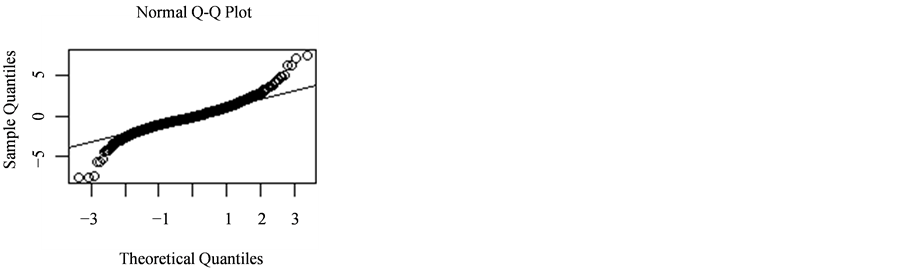Figure 1. Normal QQ plot of residuals

3. 模型诊断

3.1. 纵向子模型的残差对比

3.2. 生存子模型的残差对比(3)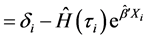(4)

Cox-Snell残差公式：(5)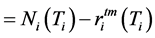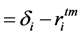(6)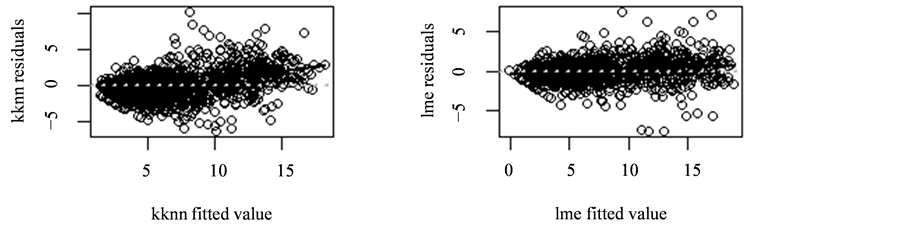Figure 2. The plot of residuals and the fitted values of the linear mixed-effect model and kknn method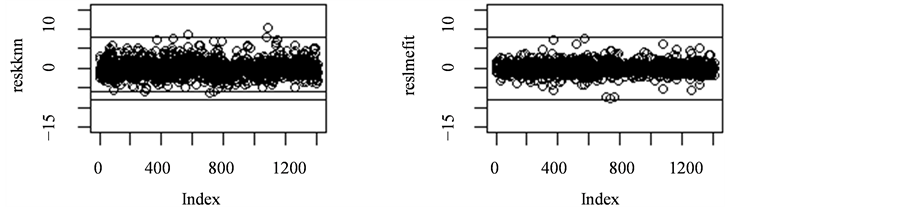Figure 3. The residuals plot of the linear mixed-effect model and kknn method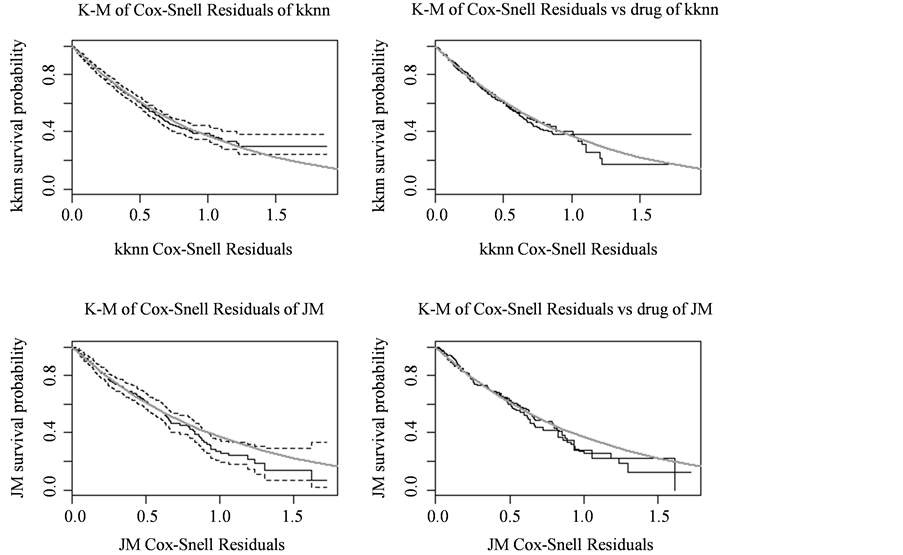Figure 4. The comparison plot of two methods

4. 结论

The Joint Model of Longitudinal and Survival Data—Based on Machine Learning Methods[J]. 统计学与应用, 2015, 04(04): 252-261. http://dx.doi.org/10.12677/SA.2015.44028

1. 1. Rizopoulos, D. (2012) Joint Models for Longitudinal and Time-to-Evwnt Data with Applications in R. Chapman &Hall/CRC Biostatistics Series, 51-155.

2. 2. Diggle, P.J., Heagerty, P., Liang, K.Y. and Zeger, S.L. (2002) Analysis of Longitudinal Data. 2nd Edition, Oxford University Press, Oxford.

3. 3. Breslow, N.E. and Clayton, D.G. (1980) Approximate Inference for Stochastic Process. Academic Press, London.

4. 4. Fattinger, K.E., Sheiner, L.B. and Verotta, D. (1995) A New Method to Explore the Distribution of Inter Individual Random Effects in Non-Linear Mixed Effects Model. Biometrics, 51, 1236-1251. http://dx.doi.org/10.2307/2533256

5. 5. Laird, N. and Ware, J.H. (1982) Random-Effects Models for Longitudinal Data. Biometrics, 38, 963-974. http://dx.doi.org/10.2307/2529876

6. 6. Hedeker, D. and Gibbons, R.D. (1994) A Random Effects Ordinal Re-gression Model for Multilevel Analysis. Biometrics, 50, 933-953. http://dx.doi.org/10.2307/2533433

7. 7. Magder, L.S. and Zeger, S.L. (1996) A Smooth Nonparametric Estimate of a Mixing Distriution Using Mixtures of Gaussians. Journal of the American Statistical Association, 91, 1141-1151. http://dx.doi.org/10.1080/01621459.1996.10476984

8. 8. Kleinman, K.P. and Ibrahim, J.G. (1998) A Semipara-metric Bayesian Approach to the Random Effects Model. Biometrics, 921-938.

9. 9. Tao, H., et al. (1999) An Estima-tion Method for the Semiparametric Mixed Effects Model. Biometrics, 55, 102-110. http://dx.doi.org/10.1111/j.0006-341X.1999.00102.x

10. 10. Cox, D. (1972) Regression Models and Life-Tables (with Discussion). Journal of the Royal Statistical Society, Series B, 187-220.

11. 11. Andersen, P. and Gill, R. (1982) Cox’s Regression Model for Counting Processes: A Large Sample Study. Annals of Statistics, 10, 1100-1120. http://dx.doi.org/10.1214/aos/1176345976

12. 12. Fleming, T.R. and Harrington, D.P. (1991) Counting Processes and Survival Analysis. Wiley, New York.

13. 13. 吴喜之. 统计学: 从数据到结论[M]. 第四版, 北京: 中国统计出版社, 2014.

14. 14. 吴喜之. 复杂数据统计方法——基于R的应用[M]. 第二版, 北京: 中国人民大学出版社, 2013.

library(JM)

w=aids[,-c(10,11,12)]

attach(w)

########################传统方法

##########纵向子模型

lmefit=lme(CD4~obstime +obstime:drug,random=~obstime| patient,data=w)

(NMSE=mean((w\$CD4-predict (lmefit,data=w))^2)/mean((w\$CD4-mean(w \$CD4))^2))

summary(lmefit)

##########生存子模型

coxfit=coxph(Surv (Time,death)~drug,data=aids.id,x=T)

summary(coxfit)

##########联合模型

jointfit=jointModel (lmefit,coxfit,timeVar=obstime,method =piecewise-PH-aGH)

summary(jointfit)

##########纵向子模型的残差QQ图及Shapiro 检验

reslmefit=residuals(lmefit)

qqnorm(reslmefit)

qqline(reslmefit)

shapiro.test(reslmefit)

#########################机器学习方法

##########分类树回归

library(rpart.plot)

cf1=rpart(CD4~.,data=w)

(NMSE=mean((w\$CD4-predict(cf1,data=w))^2)/mean((w\$CD4-mean(w\$CD4))^2))

##########Bagging回归

library(ipred)

set.seed(110)

cf2=bagging(CD4~.,data=w,coob=T,control=rpart.control(xval=10))

(NMSE=mean((w\$CD4-predict(cf2,data=w))^2)/mean((w\$CD4-mean(w\$CD4))^2))

##########随机森林回归

library(randomForest)

set.seed(110)

cf3=randomForest(CD4~.,data=w[,-1],importance=T,proximity=T)

(NMSE=mean((w\$CD4-predict(cf3,data=w[,-1]))^2)/mean((w\$CD4-mean(w\$CD4))^2))

##########最邻近方法

library(kknn)

set.seed(110)

cf4=kknn(CD4~.,train=w,test=w)

cf4fit=cf4\$fit

(NMSE=mean((w\$CD4-cf4fit)^2)/mean((w\$CD4-mean(w\$CD4))^2))

##########支持向量机

library(rminer)

set.seed(110)

cf5=fit(CD4~.,w,model=svm)

y=predict(cf5,w)

(NMSE=mean((w\$CD4-y)^2)/mean((w\$CD4-mean(w\$CD4))^2))

##########生存子模型

library(survival)

sf=coxph(Surv(Time,death)~drug+cf4fit,w)

summary(sf)

##################纵向部分残差对比

par(mfrow=c(1,2))

#######机器学习方法kknn

reskknn=w\$CD4-cf4fit

plotResid=function(x,y,col.loess=black,...){

plot(x,y,...)

lines(lowess(x,y),col=col.loess,lwd=2)

abline(h=0,lty=3,col=grey,lwd=2)}

plotResid(cf4fit,reskknn,xlab=kknn fitted value,ylab=kknn residuals)

#######线性混合效应模型

fitvalue=fitted(lmefit)

plotResid(fitvalue,reslmefit,xlab=lme fitted value,ylab=lme residuals)

plot(reskknn,ylim=c(-15,15))

abline(h=8);abline(h=-8);abline(h=-6)

plot(reslmefit,ylim=c(-15,15))

abline(h=8);abline(h=-8)

##################生存部分残差比较

par(mfrow=c(2,2))

#########kknn方法

es=residuals(sfmartingale,collapes=T)

es1=death-es#CoxSnell残差

aa=Surv(es1,death)

sfit=survfit(aa~1)

plot(sfit,mark.time=F,xlab=kknn Cox-Snell Residuals,ylab=kknn survival probability,main=K-M of Cox-Snell Residuals of kknn)

sfit1=survfit(aa~drug)

plot(sfit1,mark.time=F,xlab=kknn Cox-Snell Residuals,ylab=kknn survival probability,main=K-M of Cox-Snell Residuals vs drug of kknn)

##########线性混合效应

resCS=residuals(jointfit,process=Event,type= CoxSnell)

sfit3=survfit(Surv(resCS,death) ~ 1, data = aids.id)

plot(sfit3,mark.time=F,xlab=JM Cox-Snell Residuals,ylab=JM survival probability,main=K-M of Cox-Snell Residuals of JM)